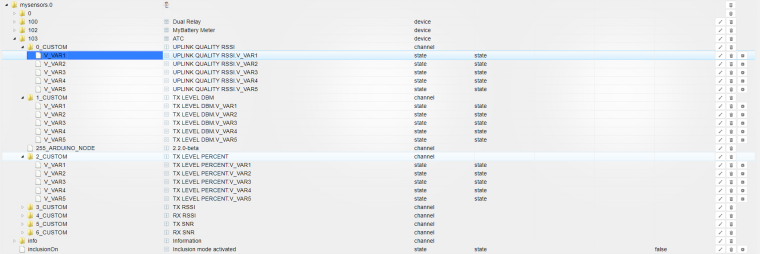# New version of the MySensors adapter

• I have just commited some changes to the iobroker MySensors Adapter on github. All set command are send with ack flag now and the states in iobroker get an ack on successful transmission, too. The new version is not being published via the iobroker admin, yet. You have to download it from github and copy it to your iobroker directory. Please test and report any problems you are facing.

• I made some test of the latest development branch 2.2.0-rc1. The RFM69_RFM95_ATC_SignalReport.ino got six Custom Values (V_CUSTOM) which are not correctly shown in ioBrocker and the values are empty.The adapter version is the 1.0.10.
Here the log of my sensor:

18 MCO:BGN:INIT NODE,CP=RPNNA---,VER=2.2.0-rc.1
28 TSM:INIT
28 TSF:WUR:MS=0
32 TSM:INIT:TSP OK
34 TSF:SID:OK,ID=103
36 TSM:FPAR
40 TSF:MSG:SEND,103-103-255-255,s=255,c=3,t=7,pt=0,l=0,sg=0,ft=0,st=OK:
929 TSF:MSG:FPAR OK,ID=0,D=1
2050 TSM:FPAR:OK
2050 TSM:ID
2052 TSM:ID:OK
2054 TSM:UPL
2062 TSF:MSG:SEND,103-103-0-0,s=255,c=3,t=24,pt=1,l=1,sg=0,ft=0,st=OK:1
2107 TSF:MSG:PONG RECV,HP=1
2111 TSM:UPL:OK
2127 TSF:MSG:SEND,103-103-0-0,s=255,c=3,t=15,pt=6,l=2,sg=0,ft=0,st=OK:0100
2183 TSF:MSG:SEND,103-103-0-0,s=255,c=0,t=17,pt=0,l=10,sg=0,ft=0,st=OK:2.2.0-rc.1
2209 TSF:MSG:SEND,103-103-0-0,s=255,c=3,t=6,pt=1,l=1,sg=0,ft=0,st=OK:0
4225 TSF:MSG:SEND,103-103-0-0,s=255,c=3,t=11,pt=0,l=3,sg=0,ft=0,st=OK:ATC
4249 TSF:MSG:SEND,103-103-0-0,s=255,c=3,t=12,pt=0,l=3,sg=0,ft=0,st=OK:1.0
4304 TSF:MSG:SEND,103-103-0-0,s=1,c=0,t=23,pt=0,l=12,sg=0,ft=0,st=OK:TX LEVEL DBM
4335 TSF:MSG:SEND,103-103-0-0,s=2,c=0,t=23,pt=0,l=16,sg=0,ft=0,st=OK:TX LEVEL PERCENT
4421 TSF:MSG:SEND,103-103-0-0,s=5,c=0,t=23,pt=0,l=6,sg=0,ft=0,st=OK:TX SNR
4446 TSF:MSG:SEND,103-103-0-0,s=6,c=0,t=23,pt=0,l=6,sg=0,ft=0,st=OK:RX SNR
4456 MCO:REG:REQ
4472 TSF:MSG:SEND,103-103-0-0,s=255,c=3,t=26,pt=1,l=1,sg=0,ft=0,st=OK:2
4507 MCO:PIM:NODE REG=1
4509 MCO:BGN:STP
4511 MCO:BGN:INIT OK,TSP=1
4528 TSF:MSG:SEND,103-103-0-0,s=0,c=1,t=48,pt=2,l=2,sg=0,ft=0,st=OK:-13
4550 TSF:MSG:SEND,103-103-0-0,s=1,c=1,t=48,pt=2,l=2,sg=0,ft=0,st=OK:-2
4575 TSF:MSG:SEND,103-103-0-0,s=2,c=1,t=48,pt=2,l=2,sg=0,ft=0,st=OK:0
4597 TSF:MSG:SEND,103-103-0-0,s=3,c=1,t=48,pt=2,l=2,sg=0,ft=0,st=OK:-31
4622 TSF:MSG:SEND,103-103-0-0,s=4,c=1,t=48,pt=2,l=2,sg=0,ft=0,st=OK:-26
4646 TSF:MSG:SEND,103-103-0-0,s=5,c=1,t=48,pt=2,l=2,sg=0,ft=0,st=OK:-256
4671 TSF:MSG:SEND,103-103-0-0,s=6,c=1,t=48,pt=2,l=2,sg=0,ft=0,st=OK:-256

Other sensor values like V_TEMP for example are represented like expected.

• @Raig I must confest I have no clue what these custom values are good for and should look like. What kind of values do you expect? Strings? Numbers?

Out of the API description for V_Custom: "Custom messages used for controller/inter node specific commands"

So it seems totally unspecified what commands or data is inside a V_Custom message.

• @Jan-Gatzke The Log Parser says the payload type is P_INT16 so I would expect a number. It is also unclear why V_VAR1 to V_VAR5 on every senor appear.

• @raig would you sage share your sketch?

2

8

1

1

20